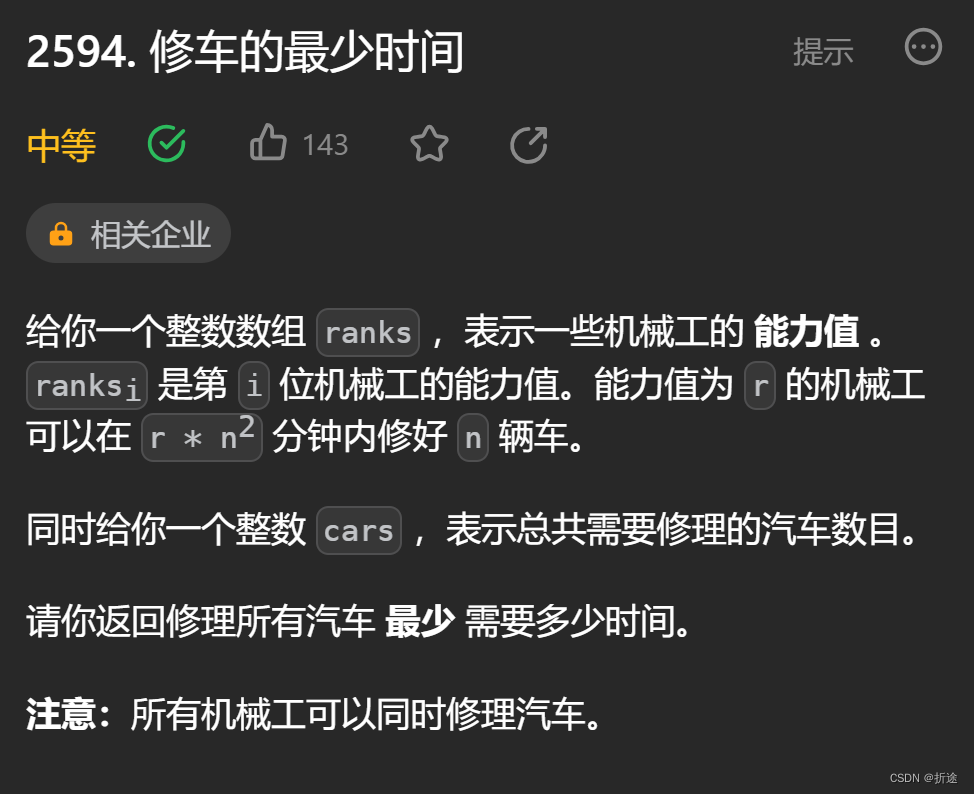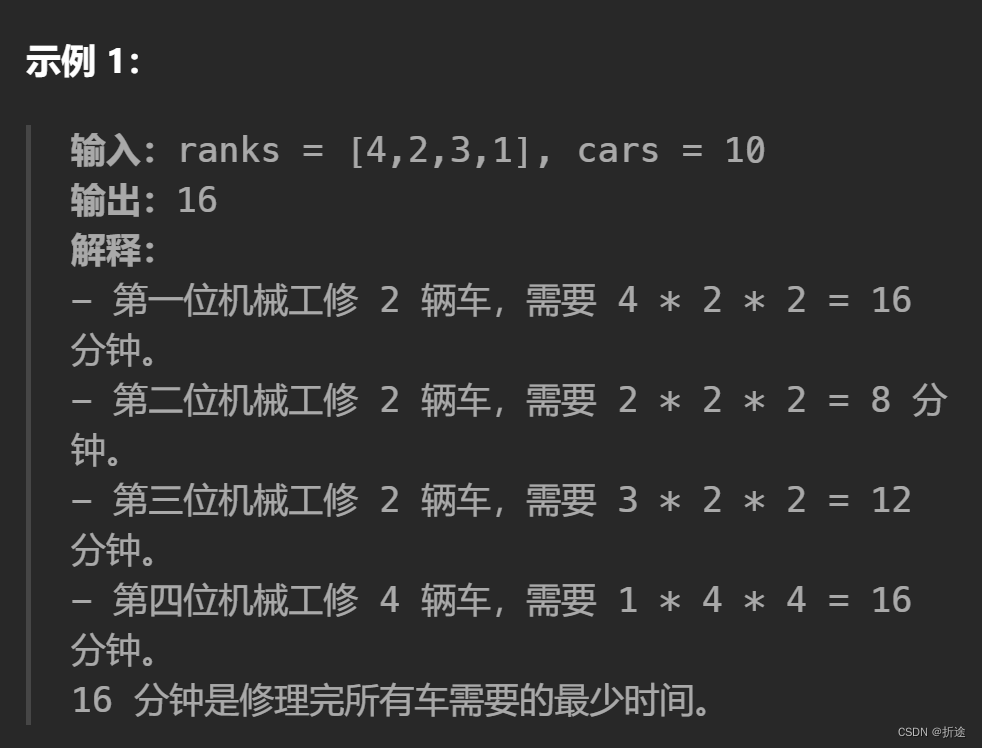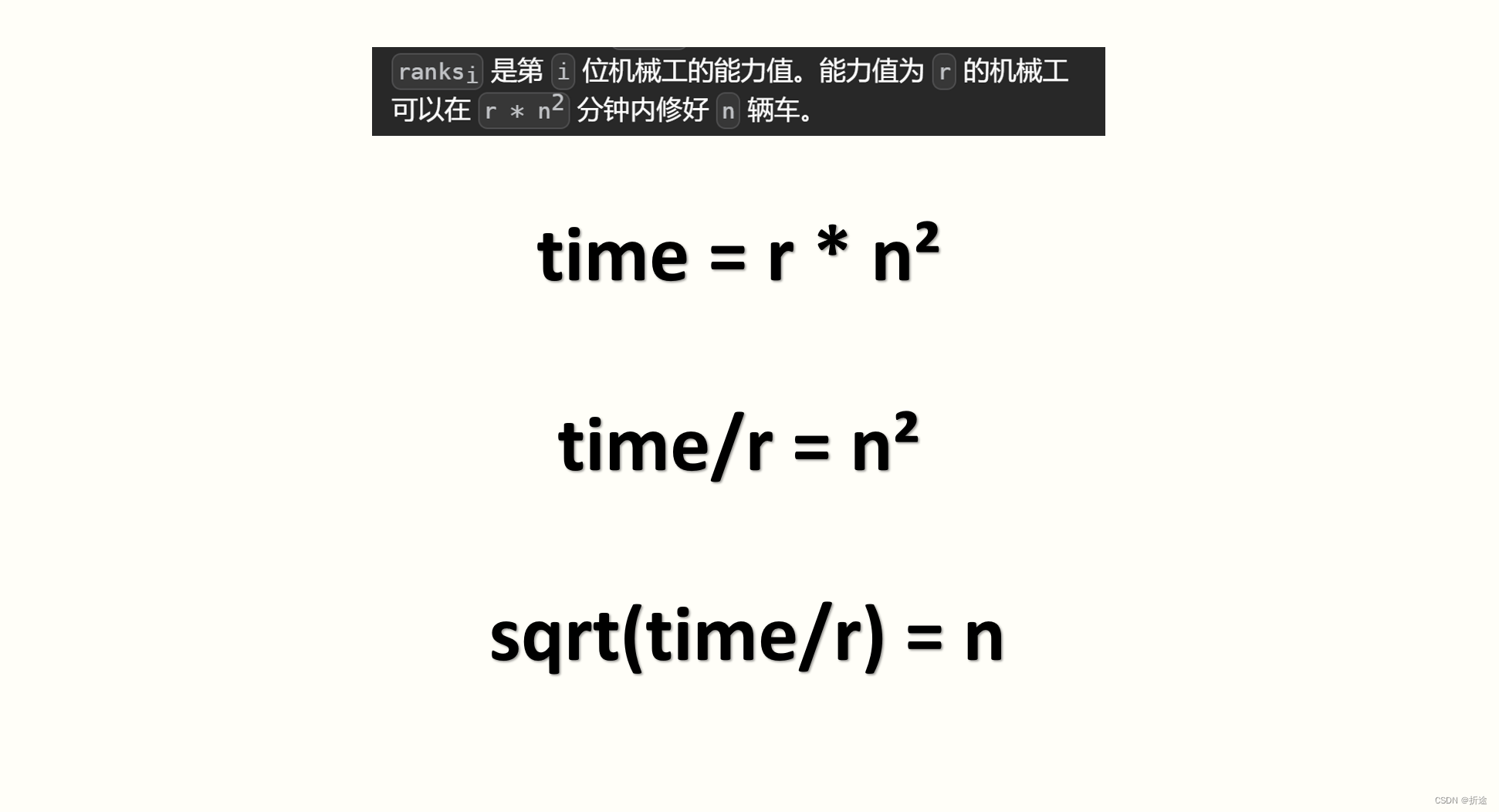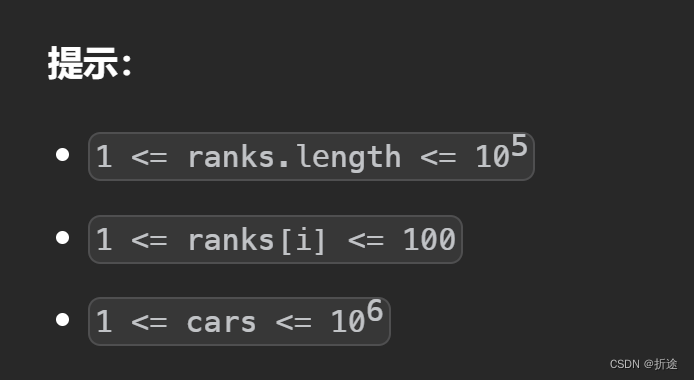# 题目：# 示例：# 分析：# 代码：

``````class Solution {
public:
bool ok(vector<int>& ranks,int cars,long long time){
long long repair=0;
for(int& rank:ranks){
repair+=static_cast<long long>(sqrt(time/rank));    //计算time的时间内可以修理多少车
}
return repair>=cars;
}
long long repairCars(vector<int>& ranks, int cars) {
long long l=1,r=LLONG_MAX;          //左闭右开,r最大为pow(10,6+6+2);
while(l<r){
long long mid=l+(r-l)/2;        //防止数值溢出的写法,等于(l+r)/2
if(ok(ranks,cars,mid)) r=mid; //如果修的车比需要的多,那么缩小右边界
else l=mid+1;                   //如果修的车比需要的少,那么缩小左边界
}
return l;
}
};``````

### 网站公告